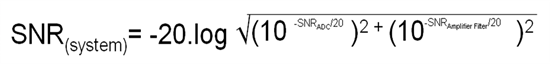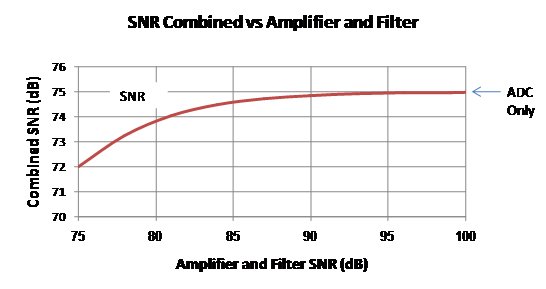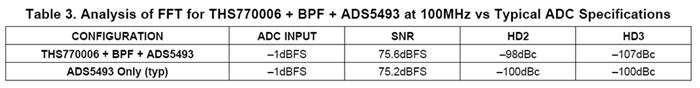# Amplifiers: Driving High Speed Analog to Digital Converters

Jim Karki, our system/applications engineer for high speed amplifier, explains:

Op amps typically specify input referred spectral noise density in nV/rtHz. SNR calculation involves first calculating the output noise based on the input noise, the gain of the amplifier, and the value of resistors used. The output spectral noise is then integrated over the bandwidth of the filter and compared to the full scale input of the ADC.

In the case of an amplifier like THS770006, (a high-speed fully fifferential ADC driver amplifier with +6dB fixed gain) it has internal resistors and fixed gain so the calculation is simplifier - the output noise is specified directly as 3.4nV/rtHz at lower frequency, which increases closer to 4nV/rtHz at 200MHz. The SNR is inversely proportional to the square root of the bandwidth, so higher bandwidth means lower SNR. For example: given FS input to ADC = 3Vpp, the equivalent SNR of the THS770006 is 96.8dB at 20MHz BW, 92.8dB at 50MHz BW, 89.8dB at 100MHz BW, and so on.

Noise or SNR is always added in RMS fashion like power. You convert the SNR dB values to linear values, square them, add together, take the square root of the sum, and covert back to dB. In equation form:The practical implication of this formula is that if the ADC and amplifier plus filter SNRs are equal, the overall SNR is 3dB less than the ADC. With higher SNR performance from the amplifier and filter, the SNR asymptotically approaches that of the ADC. 0.1dB degradation is achieved when the amplifier and filter are optimized for 15dB better SNR than the ADC.

The graph below shows the combined SNR of an ADC with SNR=75dB versus amplifier with band limiting filter SNR from 75dB to 100dB. Since noise is well behaved from a statistical point of view, the calculation is reasonably accurate, but sample to sample variations will be seen due to the random nature of noise.Another example on using differential amplifiers to drive high speed 16 bit ADCs is the THS770006 used with the ADS5493, a 16 bit 130MSPS ADC. The interface is shown in the application section of the THS770006 datasheet (page 23). In that example, a 30MHz band-pass filter at 100MHz center frequency is used and the SNR result is basically the same as the preliminary specification for the device:Of course, this is not the only ADC driver avalable - check out the other options for other configurations here. Just remember: any amplifier used for ADC input drive must have at least equal or better SNR, than the ADC itself.

• Are there any single power supply, differential amplifiers, that go down to 3 Volts?